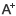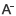# 小学一年级数学第一学期期中试题日期：2020-01-18     来源：www.zhixueshuo.com    作者：智学网    浏览：793    评论：0

期末考试了，大家要认真的复习哦，下面我们就给大家共享看看小学一年级数学，欢迎大家来阅读看看哦小学一年级上册期末考试数学试题

一、直接写得数。

2+3= 5+3= 7-3= 10-7=

14-4= 4+6= 10+9= 9-9=

9+8= 11-6= 5+9= 13-4=

3+9= 7+7= 17-8= 15-9=

10+3= 16-10= 10-0= 2+14=

10-3-7= 9-2+6= 10-7+3= 7+0+6=

二、填一填。

1.数一数，填一填。

2.18里面有个十和个一。

3.个位上是3，十位上是1的数是。

4.和10相邻的两个数是和。

5.在○里填上、或=

9○8 6+9○14 13-7○8

9+4○9-4 15-9○15- 6 5+6○6+5

6.

一共有只动物。从左边数，排第;从右边数，排第。

前面有只小动物，后面有只小动物。

7. +4 = 9 14- = 10 +=11

8. 按规律填一填。

4 6 10

□ ○ △ □ ○ △ □ ○ △

9.比一比。

下面哪种水果重? 在最高的动物下面画

在重的下面画。 ，在最矮的动物下面画○。

三、圈一圈。把每行中不一样类的圈出来。

四、选一选。右面的哪一幅照片是小明看到的?请在后面的括号里画　。

五、数一数，填一填。

个 个 个 个

六、看图列式计算。

+= +=

-= -=

七、解决问题。

1.

他们两人一共吃了多少个?

2.

吃了多少个?

3.

小红要买一个 和一本 ，一共要用多少元钱?

小军带了14元钱，买了一架 ，还剩多少元?

参考答案

一、5 8 5 3 10 10 19 0 17 5 14 9 12 14 9 6 13 6 10 16 0 13 6 13

二、1.8 17 20

2.1 8 3.13 4.9 11 5. =

6.8 6 6 4 3 7.5 4 5 6

8.2 8 12 □ ○

9.

三、

四、

五、4 4 2 2

六、

8分。 8+4=12 4+8=12 12-4=8 12-8=4

3分。 7+4=11

3分。 15-7=8

3分。 16-4-5=7

七、12分。 每式3分。

1. 8+6=14

2. 12-3=9

3.5+7=12 14-9=5

一年级上册数学期末考试题目

一、我wǒ会hu填tin。

1.数一数。

,　　,

,　　 ,

2.照样子，填一填。

3.2个十是;16里面有个十和个一;和13相邻的数是和。

4.在里填上或=。

2+912　　　　　4+812　　　　　19-78

6+915 4+815-3 10-72+7

5.在里填上+或-。

122=10 38=6+5 17-10=16

10-54=3-2 3+4+5=84 19-3-2=151

6.◇　☆　□　▲　⊙　◆　★　㊣

上图共有个图案，从右数起，□排第，⊙排第。

从左数起，▲排第，◆排第，☆后面有个图案。

7.+5=19　-7=10　15-=10

8.数一数。

长方体有个，正方体有个，圆柱有个。

二、看kn图t填tin空knɡ。

1.上图中一共有种物品。

2.的面是，的面是。

3.的边是，边是。

4.、和在这幅图的边，、和在这幅图的边。

三、我wǒ会hu画hu。

1.比一比，哪种珠子多?画。

2.

把左边的3个贝壳圈起来。从右边数的第3个贝壳是红色的，请你涂一涂。

四、算sun一yi算sun。

11+5=　　　　19-8=　　　　15-10=　　　　10+5=

12-10= 7+6= 6+9= 2+9=

19-9+4= 13-2+5= 1+6+8= 3+6+7=

5+5+6= 2+8+7= 0+6+7= 4+9-10=

五、连lin一yi连lin。

六、看kn图t列li式sh计j算sun。

1.

=

2.

=

3.

=

4.

=

七、生shēnɡ活hu中zhōnɡ的de问wn题t。

1.

一共要做多少道题?

=

2.原来地上有17只，后来爬走了6只。还剩下几只?

=

3.一共有多少片树叶?

=或 =

4.

=

小学一年级数学第一学期期中试题

一、我是神算手

容易加减

8-2= 12-1= 8+8= 7+11=

5+6= 3+13= 9-4= 19-6=

加减混合

17-3-2= 9+8-3= 8+2+7= 17-4+3=

2+3+7= 15-5-8= 16-5+8= 6+4-5=

在里填上适合的数

7+=18 -5=5 4+=9 15-=10

二、在○里填上或=

15+0○17-1 14-2○8+4 6+8○18-6

三、想一想，填一填

1、看图写数

2、12的个位上是，十位上是。

3、16是由个十和个一组成的，这个数加上就是20。

4、个位和十位上的数字都是1的数是。

5、与15相邻的两个数是和。

6、12和14中间的数是，这个数减去3就是。

7、看图数一数，填一填。

总共有人，小红前面有人，小红后面有人;从左边数起，小红在第位。

8、在□里填上适合的数。

9、看钟面写时间。

时 时 快时了 时过了

四、比一比，画一画

1、画△，比★多3个:

★ ★ ★ ★ ★ ★

2、画□，比★少7个:

★ ★ ★ ★ ★ ★ ★ ★ ★ ★ ★ ★

3、画与★一样多:

★ ★ ★ ★ ★ ★

五、我会看图解题。

1、认一认，数一数。

个 个 个 个

2、 比 多个。

3、 和 一共有个。

六、我是小法官

1、比15少3的数是11。

2、昨天是星期一，明天是星期三。

3、小明的左手边是小红，小红的右手边就是小龙。

4、2个十就是20。

5、图形 是由7个 拼成的。

七、我会解决实质问题。

1、

4、他们一共剪了多少朵窗花?

答案及评分准则

一、我是神算手

容易加减

8-2= 6 12-1= 11 8+8=16 7+11=18

5+6=11 3+13= 16 9-4= 5 19-6=13

加减混合

17-3-2=12 9+8-3=14 8+2+7=17 17-4+3=16

2+3+7= 12 15-5-8=2 16-5+8=19 6+4-5=5

在里填上适合的数

7+=18 -5=5 4+=9 15-=10

二、在○里填上或=

， =，

三、想一想，填一填

1、20，15, 14 2、2, 1 3、1, 6, 4 4、11

5、14，16 6、13，10 7、12, 3，8，9

8、4，12, 9, 17 9、6, 3，5, 9

四、比一比，画一画

五、我会看图解题。

1、4，1, 2, 4 2、3 3、6

六、我是小法官

1、 2、 3、 4、 5、

七、我会解决实质问题。

1、8 2、11 3、4 4、16 5、14

0相关评论